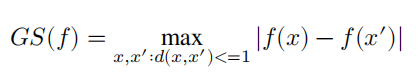# Programming Differential Privacy 阅读参考（part 3）

Programming Differential Privacy 链接

## ch6 敏感度• d(x,x')：数据集x和x'之间的距离，如果两个数据集之间的距离<=1，就认为它们是相邻/临近的（neighbors）

### 距离

d(x,x')=|x-x'∪x'-x|


## ch7 近似差分隐私

• 隐私参数δ：表示失败概率
• 我们有1-δ的概率获得纯粹差分隐私（pure differential privacy）的保护，即Pr[F(x)=S] / Pr[F(x')=S] <= eε
• 通常要求δ是一个很小的数，一般小于1/n2，其中n是数据库的大小
• 失败的时候并不会造成整个数据库泄露的严重后果，实际上是温和地（gracefully）失败

### 近似差分隐私的性质

• 如果F1 (x)满足(ε11)-DP，F2 (x)满足(ε22)-DP
• 则同时发布两个结果的机制G(x)=(F1 (x), F2 (x))满足(ε12 , δ12)-DP

### 高斯机制

F(x) = f(x) + N(σ2)，其中σ2 = 2s2log(1.25/δ) / ε2

• s是f的敏感度
• N(σ2)表示均值为0，方差为σ2的高斯（正态）分布的采样结果
• log x表示自然对数，即ln x

### 向量值函数及其敏感度

• L1范数（L1 norm）：向量中各个元素的和。二维空间中，两个向量之差的L1范数就是曼哈顿距离
• L2范数（L2 norm）：向量中各个元素平方和的平方根。二维空间中，两个向量之差的L2范数就是欧氏距离
• L2范数总是小于等于L1范数

• L1敏感度：GS(f) = maxd(x,x')<=1 ||f(x)-f(x')||1 ，L1敏感度等于向量中各个元素敏感度的和
• L2敏感度：GS(f) = maxd(x,x')<=1 ||f(x)-f(x')||2
• 对于长向量，L2敏感度比L1敏感度低得多

### 高级组合性

(ε,δ)-DP引入了一种DP机制串行组合性的新分析方法，可以进一步降低隐私消耗

k-折叠适应性组合（k-fold adaptive composition）：一系列机制m1, m2, ... ,mk组合起来，每个机制满足

• 适应性：可以根据之前所有机制m1, m2, ... ,mi-1的输出，选择下一个机制mi
• 组合性：每个机制mi的输入既包括隐私数据集，也包括previous机制的所有输出

• 如果k-折叠适应性组合m1, m2, ... ,mk中的每一个机制mi都满足ε-DP
• 则对任意δ’>=0，整个 k-折叠适应性组合满足 (ε',δ')-DP，其中 ε' = 2ε√2klog(1/δ')

### 近似差分隐私的高级组合性

• 如果k-折叠适应性组合m1, m2, ... ,mk中的每一个机制mi都满足 (ε,δ)-DP
• 则对任意δ’>=0，整个 k-折叠适应性组合满足 (ε',kδ+δ')-DP，其中 ε' = 2ε√2klog(1/δ')

posted @ 2022-11-06 10:51  CCchaos  阅读(133)  评论(0编辑  收藏  举报